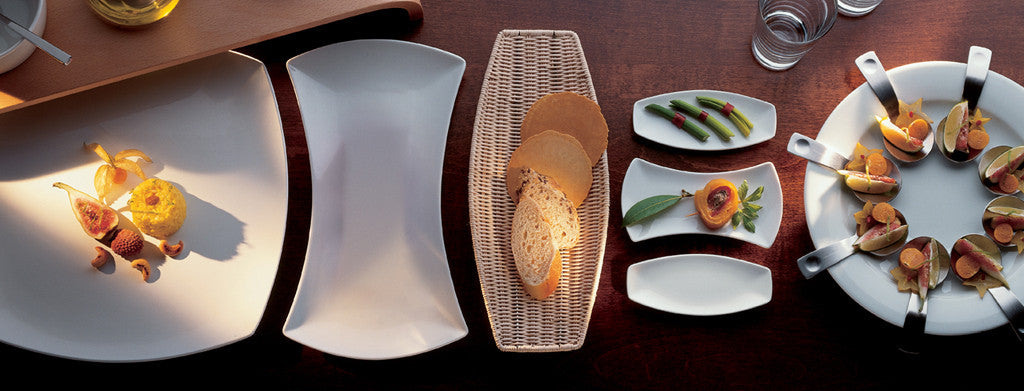Shopping Cart

### Refine

View all

#### Price# Crockery£9.29 each (ex VAT)£7.15 each (ex VAT)£4.92 each (ex VAT)£3.72 each (ex VAT)£5.29 each (ex VAT)£10.85 each (ex VAT)£8.49 each (ex VAT)£7.89 each (ex VAT)£16.29 each (ex VAT)£11.69 each (ex VAT)£9.49 each (ex VAT)£5.69 each (ex VAT)£26.39 each (ex VAT)£13.89 each (ex VAT)£6.09 each (ex VAT)£16.69 each (ex VAT)£13.29 each (ex VAT)£33.89 each (ex VAT)£16.99 each (ex VAT)£8.69 each (ex VAT)£21.49 each (ex VAT)£20.69 each (ex VAT)£16.49 each (ex VAT)£13.89 each (ex VAT)£8.39 each (ex VAT)£7.19 each (ex VAT)£4.79 each (ex VAT)£12.31 each (ex VAT)£9.88 each (ex VAT)£8.39 each (ex VAT)£8.57 each (ex VAT)£7.89 each (ex VAT)£5.75 each (ex VAT)£3.99 each (ex VAT)£5.29 each (ex VAT)£4.73 each (ex VAT)£3.45 each (ex VAT)£6.00 each (ex VAT)£3.51 each (ex VAT)£18.47 each (ex VAT)£16.28 each (ex VAT)£10.13 each (ex VAT)£9.45 each (ex VAT)£8.43 each (ex VAT)£7.62 each (ex VAT)£2.70 each (ex VAT)£4.42 each (ex VAT)£3.41 each (ex VAT)